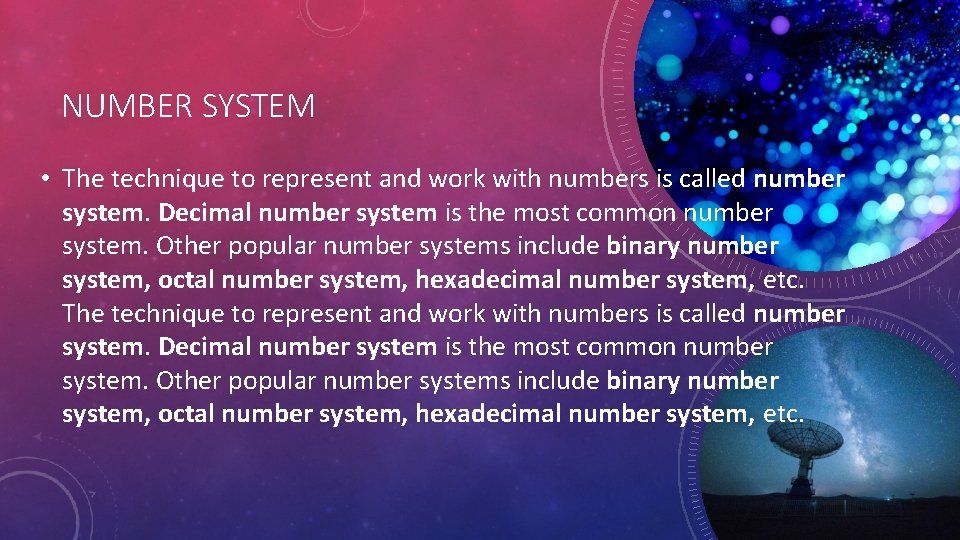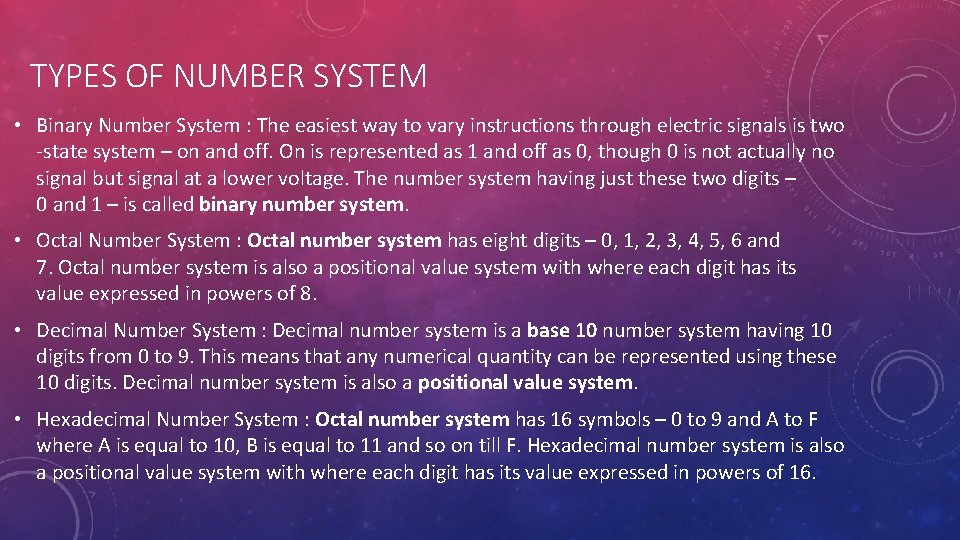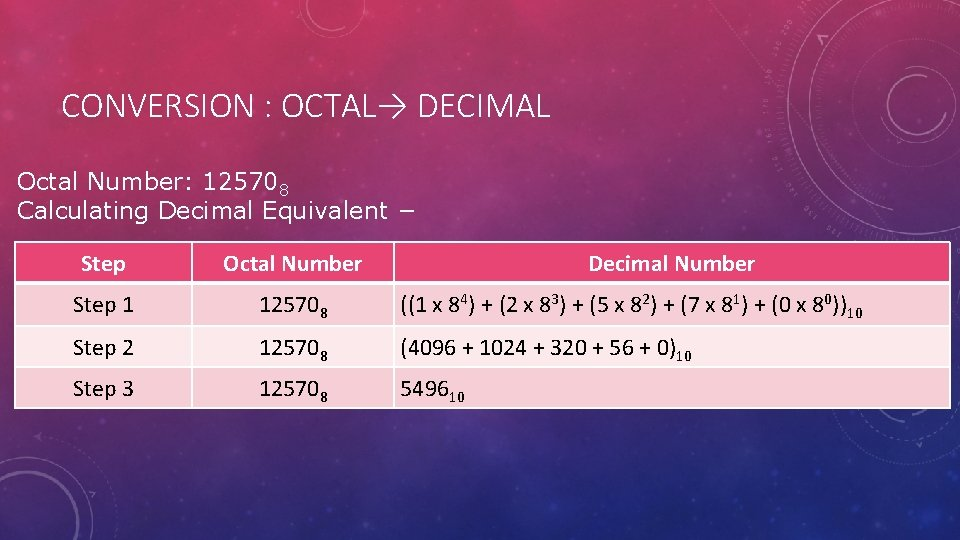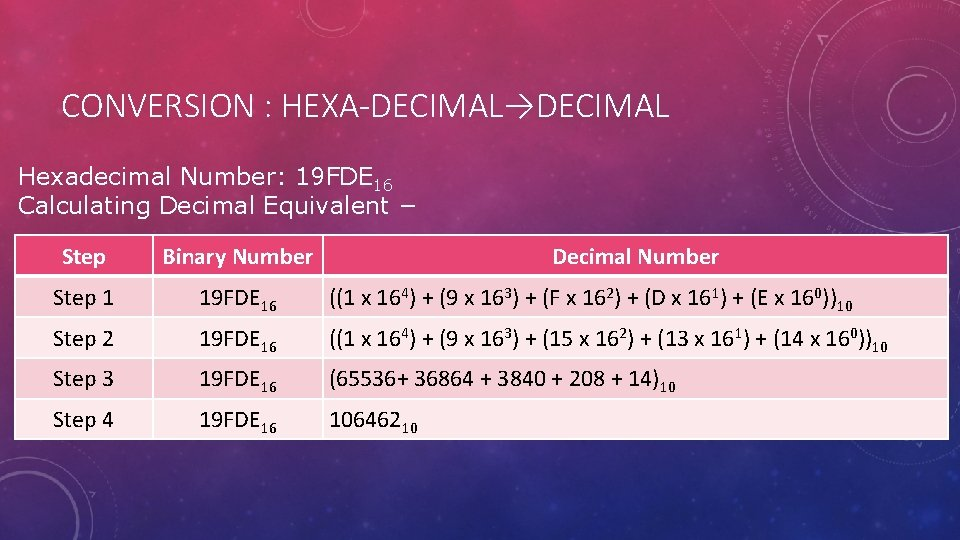# NUMBER SYSTEM NUMBER SYSTEM The technique to represent

• Slides: 11NUMBER SYSTEMNUMBER SYSTEM • The technique to represent and work with numbers is called number system. Decimal number system is the most common number system. Other popular number systems include binary number system, octal number system, hexadecimal number system, etc.TYPES OF NUMBER SYSTEM • Binary Number System : The easiest way to vary instructions through electric signals is two -state system – on and off. On is represented as 1 and off as 0, though 0 is not actually no signal but signal at a lower voltage. The number system having just these two digits – 0 and 1 – is called binary number system. • Octal Number System : Octal number system has eight digits – 0, 1, 2, 3, 4, 5, 6 and 7. Octal number system is also a positional value system with where each digit has its value expressed in powers of 8. • Decimal Number System : Decimal number system is a base 10 number system having 10 digits from 0 to 9. This means that any numerical quantity can be represented using these 10 digits. Decimal number system is also a positional value system. • Hexadecimal Number System : Octal number system has 16 symbols – 0 to 9 and A to F where A is equal to 10, B is equal to 11 and so on till F. Hexadecimal number system is also a positional value system with where each digit has its value expressed in powers of 16.BINARY NUMBER SYSTEM • Characteristics of the binary number system are as follows − • Uses two digits, 0 and 1. • Also called as base 2 number system. • Each position in a binary number represents a 0 power of the base (2). Example 20 • Last position in a binary number represents a x power of the base (2). Example 2 x where x represents the last position - 1.OCTAL NUMBER SYSTEM • Characteristics of the octal number system are as follows − • Uses eight digits, 0, 1, 2, 3, 4, 5, 6, 7. • Also called as base 8 number system. • Each position in an octal number represents a 0 power of the base (8). Example 80 • Last position in an octal number represents a x power of the base (8). Example 8 x where x represents the last position – 1.DECIMAL NUMBER SYSTEM • Characteristics of the decimal number system are as follows − • Uses ten digits, 0, 1, 2, 3, 4, 5, 6, 7, 8, 9. • Also called as base 10 number system. • Each position in a decimal number represents a 0 power of the base (10). Example 100 • Last position in a decimal number represents a x power of the base (10). Example 8 x where x represents the last position - 1.HEXA-DECIMAL NUMBER SYSTEM • Characteristics of hexadecimal number system are as follows − • Uses 10 digits and 6 letters, 0, 1, 2, 3, 4, 5, 6, 7, 8, 9, A, B, C, D, E, F. • Letters represent the numbers starting from 10. A = 10. B = 11, C = 12, D = 13, E = 14, F = 15. • Also called as base 16 number system. • Each position in a hexadecimal number represents a 0 power of the base (16). Example, 160 • Last position in a hexadecimal number represents a x power of the base (16). Example 16 x where x represents the last position - 1CONVERSION : BINARY → DECIMAL Binary Number: 101012 Calculating Decimal Equivalent − Step Binary Number Decimal Number Step 1 101012 ((1 x 24) + (0 x 23) + (1 x 22) + (0 x 21) + (1 x 20))10 Step 2 101012 (16 + 0 + 4 + 0 + 1)10 Step 3 101012 2110CONVERSION : OCTAL→ DECIMAL Octal Number: 125708 Calculating Decimal Equivalent − Step Octal Number Decimal Number Step 1 125708 ((1 x 84) + (2 x 83) + (5 x 82) + (7 x 81) + (0 x 80))10 Step 2 125708 (4096 + 1024 + 320 + 56 + 0)10 Step 3 125708 549610CONVERSION : HEXA-DECIMAL→DECIMAL Hexadecimal Number: 19 FDE 16 Calculating Decimal Equivalent − Step Binary Number Decimal Number Step 1 19 FDE 16 ((1 x 164) + (9 x 163) + (F x 162) + (D x 161) + (E x 160))10 Step 2 19 FDE 16 ((1 x 164) + (9 x 163) + (15 x 162) + (13 x 161) + (14 x 160))10 Step 3 19 FDE 16 (65536+ 36864 + 3840 + 208 + 14)10 Step 4 19 FDE 16 10646210THANK YOU! TANMAY MUDGAL